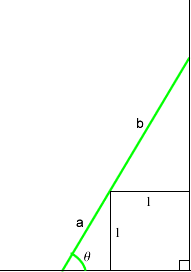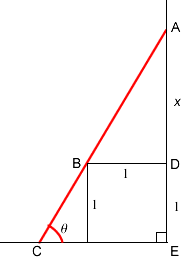#### You may also like### Golden Thoughts

Rectangle PQRS has X and Y on the edges. Triangles PQY, YRX and XSP have equal areas. Prove X and Y divide the sides of PQRS in the golden ratio.### From All Corners

Straight lines are drawn from each corner of a square to the mid points of the opposite sides. Express the area of the octagon that is formed at the centre as a fraction of the area of the square.### Star Gazing

Find the ratio of the outer shaded area to the inner area for a six pointed star and an eight pointed star.

# Slippage

##### Age 14 to 16 Challenge Level:

It is often too easy to dive into algebra or trigonometry to solve problems when other routes to solutions are available, especially when the question says "find" rather than "calculate". The two solutions below, one from Richard and one from Andrei take different approaches. We also solved this using dynamic geometry software.

Peter of Richard Hale School, approached the problem using trial and improvement. Here is his solution:

My method of trial and improvement for this problem was to pick an angle to the horizontal, consider a ladder placed at that angle and make it touch the ground, the wall and the corner of the storage box. I then calculated the length of that ladder. I then altered the angle until the length of the ladder was $3$ metres. I then used the angle to calculate the height at which the ladder touched the wall. \par This can be done by applying $\sin$ and, $\cos$ in the two right-angled triangles formed between the box, the ladder, the ground and the wall. \par Once I knew the angle at which the ladder would be $3$m long, I could apply $\sin$ in the large right-angled triangle to find the height against the wall.In the lower triangle, if we specify an angle to the horizontal $$\theta and use the 1m side of the block as a side of the triangle, we can work out the hypotenuse$$a= \frac{1}{\sin \theta}$$For the top triangle, the hypotenuse is given by$$b= \frac{1}{\cos \theta}$$. In both cases 1 is the length of the side of the box. Therefore it is possible to create one formula that can be used to easily calculate the length of the ladder from any angle to the horizontal specified. That formula, with distance as s and the angle to the horizontal as theta, is:$$s = \frac{1}{\sin \theta} + \frac{1}{\cos \theta}$$Before doing my trial and improvement, I attempted to rearrange the equation to make theta the subject. I was not able to do this, and so went ahead with my trial and improvement approach. I started with 45 degrees, which produced a ladder length of 2.828 metres. I tried the following angles, which produced the lengths shown: 50 degrees - 2.861m 60 degrees - 3.155m 55 degrees - 2.964m 56 degrees - 2.995m 57 degrees - 3.028m 56.5 degrees - 3.011m 56.25 degrees - 3.003m 56.2 degrees - 3.001m At that point I decided that the number of decimal places was enough, and that because I could not solve the equation for the right variable, it would take too long to reach the answer exactly by trial and improvement. Therefore, the ladder is placed at 56.2 degrees to the horizontal, and, using \sin ratio in the larger triangel I obtained:$$h = 3\times \sin 56.2^\circ = 2.493\mbox{m}$$On the other hand Andrei of Tudor Vianu College took an algebraic approach. Andrei ended with a quartic and offers three solutions. There is quite a jump from the ratio formula to the three solutions but the first part is very useful. I see first that in the absence of the square obstacle (in the section) the ladder could be moved continuously, and, as a limit, it could attain the height of 3 m, equal to its length. With the obstacle, it could not touch 3m height, but in any case, it must not cross the vertex of the square. A possible procedure to put the ladder against the wall is to put is vertically, near the corner of the obstacle, and to rotate it around this corner up to the moment it touches simultaneously the ground and the wall. The maximum height is obtained in the situation it touches these 3 points. This way, the problem reduces to finding the maximum of a leg of a right- angled triangle, of hypotenuses 3 m, which contains a square of side 1 m.This way the similarity ratio of the upper triangle and the whole triangle is:$$\frac{AB}{AC}=\frac{AD}{AE}$$Which gives:$$ \frac{\sqrt{x^2+1}}{3}= \frac{x}{x+1}

This could be treated as an equation in $x$. Evidently, $x$ must be positive ( being a length) and smaller than $2$ (the leg of any right angled triangle must be smaller than the hypotenuses), i.e. $x + 1 < 3$.

The equation above has three solutions for $x$:

\begin{eqnarray} x_1 &=& -\frac{1}{2}+\sqrt{\frac{5}{2}}-\frac{1}{2}\sqrt{7-\sqrt{10}} &\cong 0.6702\\ x_2 &=& -\frac{1}{2}+\sqrt{\frac{5}{2}}+\frac{1}{2}\sqrt{7-\sqrt{10}} &\cong 1.4921\\ x_3 &=& -\frac{1}{2}-\sqrt{\frac{5}{2}}-\frac{1}{2}\sqrt{7-\sqrt{10}} &\cong -3.9062 \end{eqnarray} and solution no. $3$ is not a solution for the geometry problem.

Now, from the first two solutions, the second is to be chosen, being greater, and the height corresponding to it is $x + 1 = 2.49$ m, this being the maximum height.

It seems surprising that there are only two solutions where the ladder touches the obstacle, the ground and the wall.